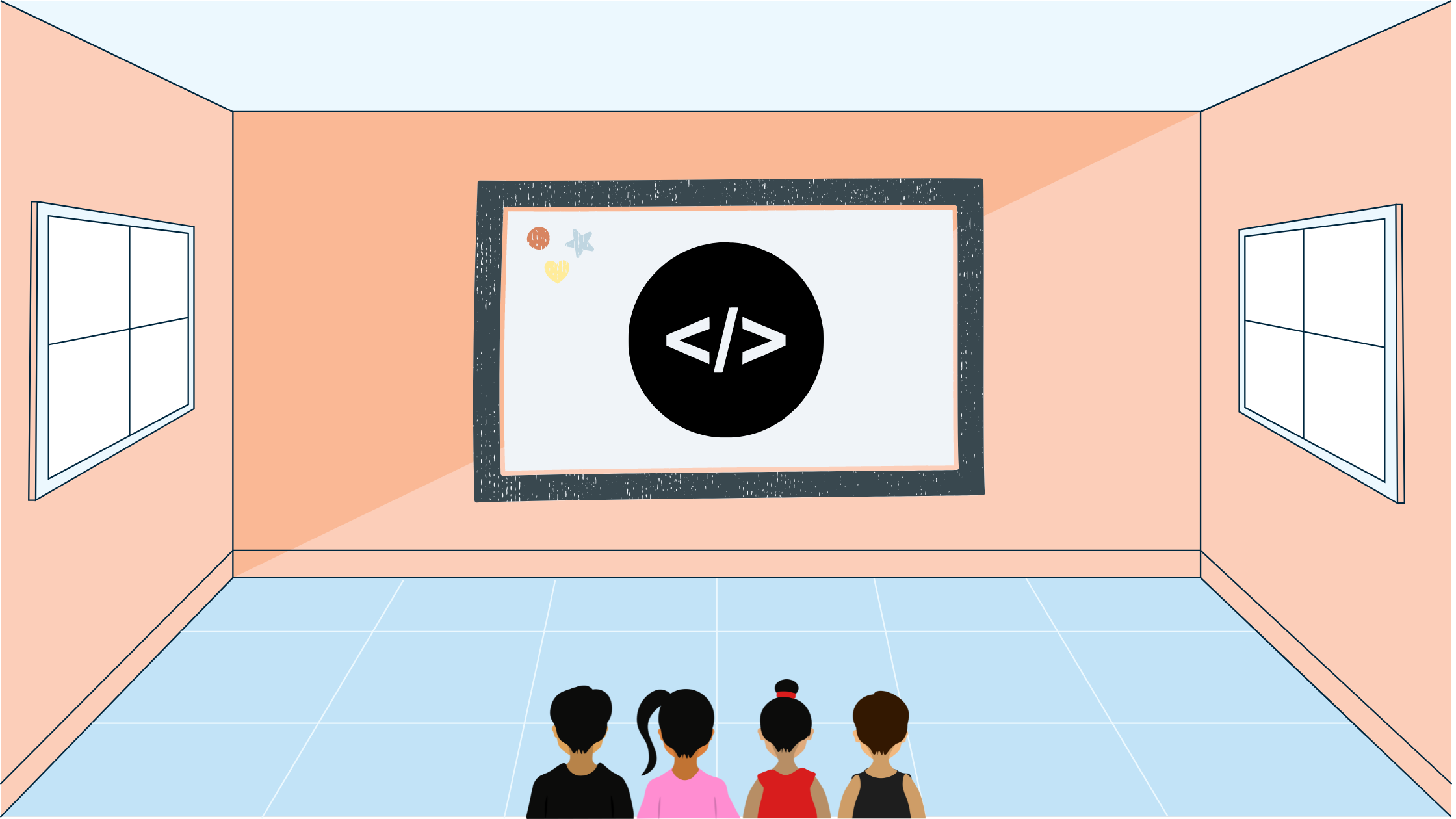Yash Singh# USACO Hurdles

In this blog post I'll go over the USACO Blocks Bronze January 2022 Problem 1 using other resources and solutions.

## Statement

We are given two lists of three words, with the first list being the answers and the second list being the guess to the answers. We knew to output the number of letters marked as green or correct and mark all other guessed letters that exist in the second list but are in the wrong position as yellow.

## Solution

We can keep a hashmap mapping a character to its frequency. Then, we can iterate through the guessed list and increment a green counter when it matches with the answer list. We can also decrement the frequency in the hashmap. Otherwise, if the answer list contains the character and the hashmap has a frequency of $>0$, then we can increment a yellow counter and decrement the guessed character from the hashmap.

However, if we try to implement and submit this solution, it would not work because if we have a list that has a bunch of character that should be marked with a yellow followed by a character that should be marked with a green, there will be a character marked with a yellow which shouldn't be so. Taking a snippet from the statement:

More precisely, if there are $x$ cows of a certain breed in the guess grid and $y cows of this breed in the answer grid (not counting cows in the right place that lead to green highlights), then only $y$ of the $x$ cows in the guess grid should be highlighted yellow.

Notice the part where it says "...not counting cows in the right place that lead to green highlights..." meaning that $y$ should not count the squares in the answer grid that are guessed correctly in the guess grid. Here is an example where our current solution fails:

ABBBA # answer
BABAA # guess

Our current solution would return three yellows and two greens, however the correct answer is two yellows and two greens. This is because, there is only one A in the answer grid that doesn't match with its corresponding guess. That means only one of the two guesses for an A in the guess grid would correspond to that A answer.

The way we can fix our solution for this problem is to iterate through all the letters and increment the green counter and decrement corresponding hashmap values first, and then look for yellows in a second iteration of all the letters.

## Implementation

Let's go through an implementation in C++:

#include <bits/stdc++.h>

using namespace std;

int main() {
ios_base::sync_with_stdio(false);
cin.tie(nullptr);
// Take in real and guess
char real;
char guess;
map<char, int> m;
for (int i{0}; i < 3; ++i) {
for (int j{0}; j < 3; ++j) {
cin >> real[i][j];
++m[real[i][j]];
}
}
for (int i{0}; i < 3; ++i) {
for (int j{0}; j < 3; ++j) {
cin >> guess[i][j];
}
}
// Increase greens
int g{0}, y{0};
for (int i{0}; i < 3; ++i) {
for (int j{0}; j < 3; ++j) {
if (real[i][j] == guess[i][j]) {
++g;
--m[real[i][j]];
}
}
}
// Now look for yellows
for (int i{0}; i < 3; ++i) {
for (int j{0}; j < 3; ++j) {
if (m[guess[i][j]] > 0 && real[i][j] != guess[i][j]) {
++y;
--m[guess[i][j]];
}
}
}
cout << g << endl << y << endl;
}

## Lessons Learnt

One lesson this problem taught us is that whenever you are missing out on a test case or two, try to list out the expectations of the statement and make sure your solution matches it.